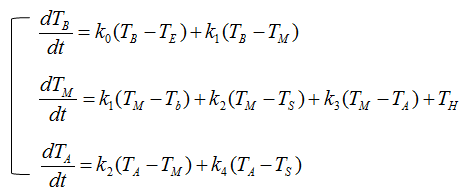DE - Modeling - Cooling                                                              Home : www.sharetechnote.com In this section, I will show you some of the examples of building differential equations for cooling & heating. As I mentioned in Governing Equation page, the most important step for cooling/heating case as well is to figure out proper governing equation (governing law). The fundamentals of Cooling problem is based on Newton's Law of Cooling. (I will add some comments on this in my own words later when I have time, but for now I would suggest you to google some basics of Newton's Law of Cooling if you are not familiar with the concept).   Cooling with No Temperature input   This example can be the most simplest case of Cooling problem and it would show you the essense of Newton's Law of cooling. The situation can be illustrated as shown below. Let's assume that you have a hot drink (like hot coffee) and put it on the table and put a thermometer in it and let it alone for several hours. You would know (from experience) that the temperature will gradually decrease. This example would explain how the temperature would change.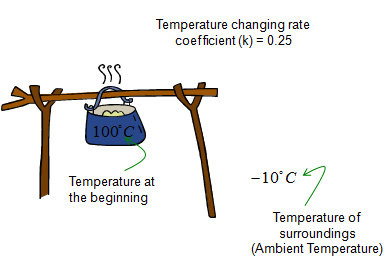The governing equation for this case can be illustrated as below. Try to follow each and every parts including all the arrows in this illustration and comments, and you would automatically get a differential equation for the situation.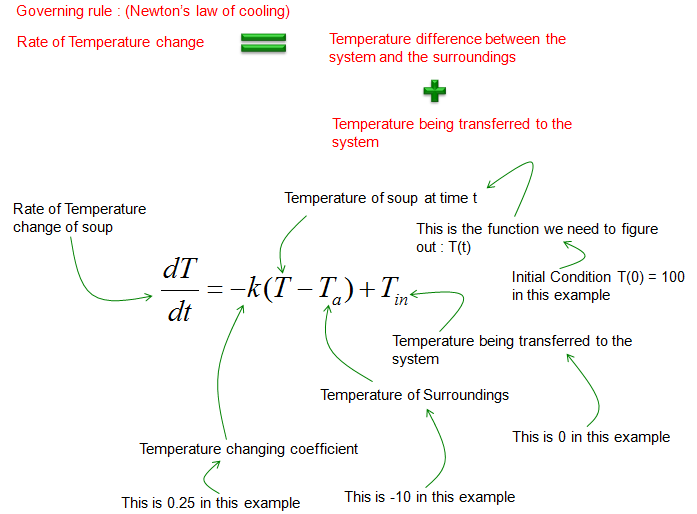If you just convert the governing law shown above into a matehmatical form, you would get the differential equation as shown below.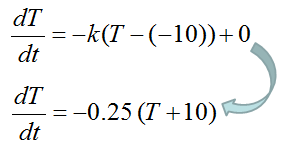Cooling with Temperature input   This example is just a little extension to previous example. In this situation, a simple heat source is added. So you would have two factors influencing on the system. One factor is removing heat (cooling) and the other factor is adding heat (heating). The situation can be illustrated as shown below.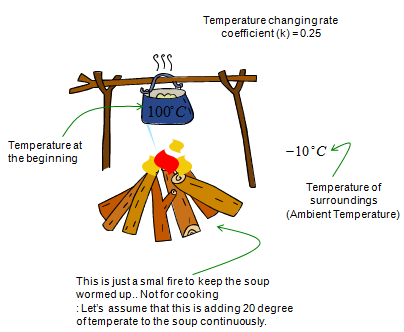The governing equation for this case can be illustrated as below. Try to follow each and every parts including all the arrows in this illustration and comments, and you would automatically get a differential equation for the situation.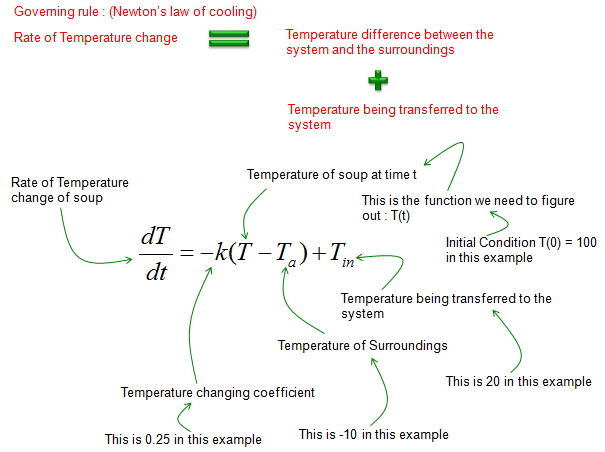If you just convert the governing law shown above into a matehmatical form, you would get the differential equation as shown below.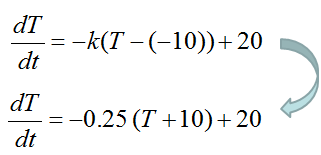Home Heating   This example would show you a more realistic case but would look much more complicated than the examples shown above. However, don't get scared of it just by the complexity. However complicated a situation look, you can always break the situation into several component which is simple enough for you to attack. Once you get the several simple blocks, just figure out the governing equation for each of the simple block and figure out the differential equation for it. When you complete building the differential equation for all the simpler component blocks, you can simply put all those equations together and get a complete system equation.   The situatioin we have to solve is to deduce the mathematical model to represent the temperature over time inside a house. It can be illustrated as shown below. This house is made up of several sectors - Basement, Main Floor,Attic and Surrounding Air. Each of these components has a couple of boundaries with the other components and each of the boundary has different heat tranfer rate.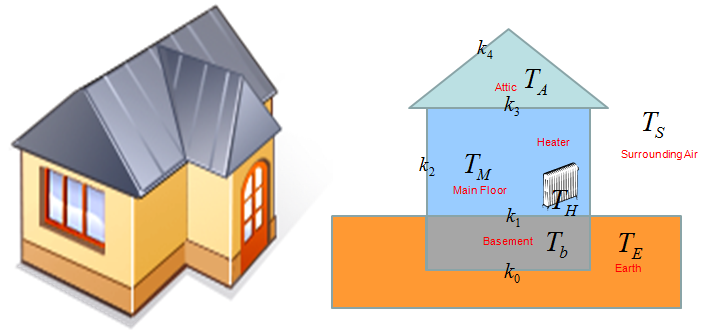First, let's deduce the differential equation for the basement. It can be illustrated as shown below. Try to follow each and every parts including all the arrows in this illustration and comments, and you would automatically get a differential equation for the situation.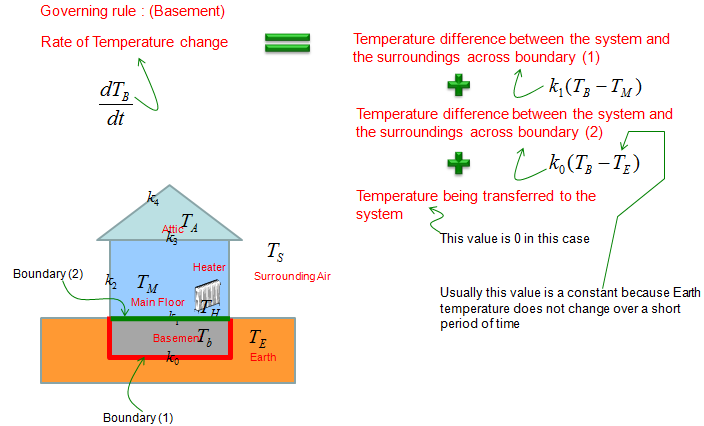If you combine all the factors described in the illustration into an equation, you would get a differential equation as shown below.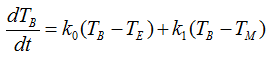Next, let's deduce the differential equation for the Main Floor. It can be illustrated as shown below. Try to follow each and every parts including all the arrows in this illustration and comments, and you would automatically get a differential equation for the situation.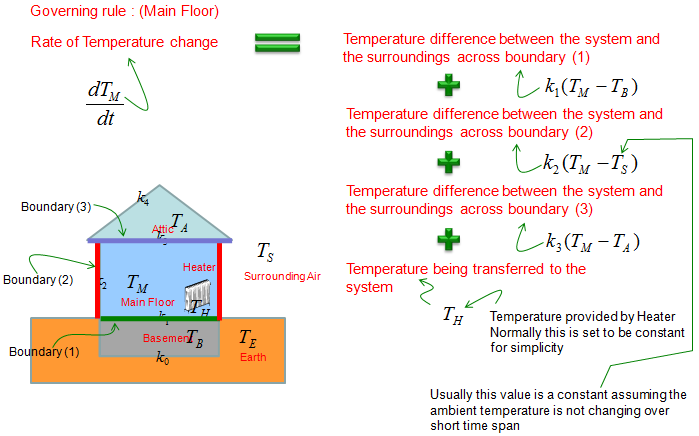If you combine all the factors described in the illustration into an equation, you would get a differential equation as shown below.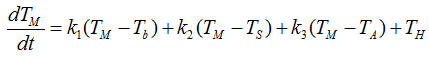First, let's deduce the differential equation for the Attic. It can be illustrated as shown below. Try to follow each and every parts including all the arrows in this illustration and comments, and you would automatically get a differential equation for the situation.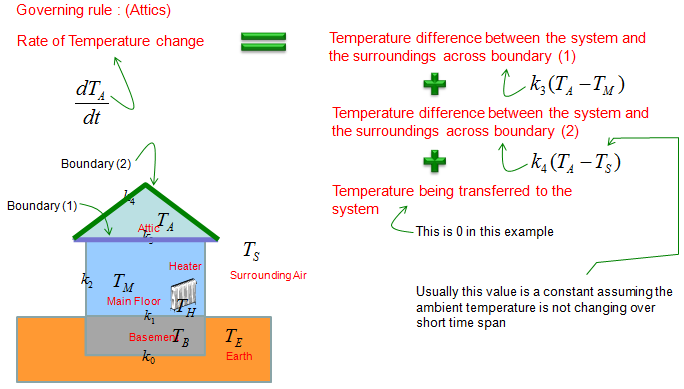If you combine all the factors described in the illustration into an equation, you would get a differential equation as shown below.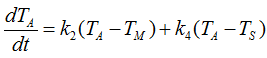Now if you just put together all the individual equations for each individual component, you would get a system equation (simultaneous equation) as shown below.